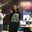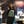Trusted answers to developer questions
Trusted Answers to Developer Questions

Related Tags

theoretical cs

# What is the Church-Turing thesis?Ayesha Kanwal

Grokking Modern System Design Interview for Engineers & Managers

Ace your System Design Interview and take your career to the next level. Learn to handle the design of applications like Netflix, Quora, Facebook, Uber, and many more in a 45-min interview. Learn the RESHADED framework for architecting web-scale applications by determining requirements, constraints, and assumptions before diving into a step-by-step design process.

## Overview

In 1936, Alan Turing presented a theoretical model for machines, or presently known as Turing machines. We use this machine to manipulate the symbols of input strings on the tape.

We describe a Turing machine as an abstract representation of a computing device.

In the same year, Alonzo Church developed a lambda calculus methodA system of mathematical logic used for expressing computation based on abstraction. It is used for the simulation of a Turing machine.. Encoding of natural numbers was introduced and called Church numerals.

### Church-Turing thesis

Alan Turing gave the idea for logical computing machines that worked on Turing expressions.

A mechanical method was made for this. Assume this method is $M$, and we use it to manipulate strings by logic and mathematics.

#### Requirements for the Church-Turing thesis

This defined method $M$ should pass the following:

• It shouldn't require any complex processing requirements.
• We obtain output after performing a finite number of steps.
• We can implement the method $M$ in the real world.
• The number of instructions for $M$ should be finite.

After considering these requirements, Church suggested a hypothesis called the Church-Turing thesis, defined below:

The assertion that partial recursive functions can be used to identify the intuitive concept of computable functions.

Despite this hypothesis, we can't prove this claim.

We describe this thesis under two main categories:

• Church-Turing thesis for decidability problems: A decidability problemA problem that is either true or false. can be solved effectively if there exists a Turing machine that halts for all of its input strings and calculates the solution.
• Extended Church-Turing thesis for decidability problems: A decidability problem is partially solvable if there exists a Turing machine that accepts the elements of the problem whose answer is "yes."

#### Proof for recursive functions

We can compute recursive functions by considering the following assumptions:

• All functions must be computable.
• Assume a computable function $f$. After performing elementary operations, the function will transform into a new function $g$. $g$ is automatically a computable function.

### Applications of the thesis

This thesis finds its applications in many calculable and computational fields. Some of these are:

• Lambda calculus
• Single and multiple tape Turing machines
• Counter machine model
• Register machine, a machine similar to the computer
• Markov algorithms
• Combinatory logic
• Pointer machines

RELATED TAGS

theoretical cs

CONTRIBUTORAyesha Kanwal